# Chapter 7 - Writing functions in Python, importing a module¶

Until now we have regarded a function as a “black box”. We put in some arguments, and we get a return value (or possibly a side-effect like output) that is produced by the function gnomes.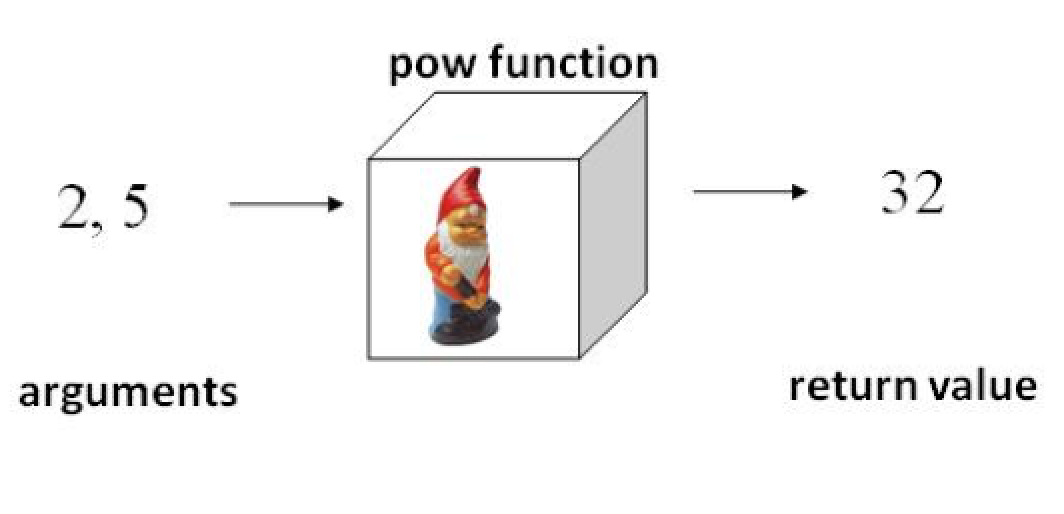Now it is time for us to go inside the black box and learn how to write our own functions in Python.

## Function parameters¶

We need variables to describe what a function does. For example, think about the pow function for computing powers. We could describe it like this:

“The pow function returns the value of one number raised to the power of another number”

But that doesn’t really describe the function very well, because when we call ( pow(2, 5) ), we need to know which number is the base and which is the exponent. So a more accurate description would be:

“the function pow(x, y) returns the value of x to the power y “

The thing to notice is that the variables x and y that we use in this description are not actually arguments to the function. They don’t have actual values. They are just “placeholders” that are used in the description of what the function does. We could just as well have said,

“the function pow(b, e) returns the value of b to the power e ”

or

“the function pow(fred, george) returns fred to the power george “

In all three cases, we’re describing exactly the same function, as shown below:These placeholder variables are called parameters. Remember that when we call a function we have to provide the actual values that we want it to use in computing the result. These values are called arguments. The way it works is that

1.the expressions we provide as arguments are evaluated,

2.those values are substituted for the parameters, and then

3.the result is produced by the function gnomes.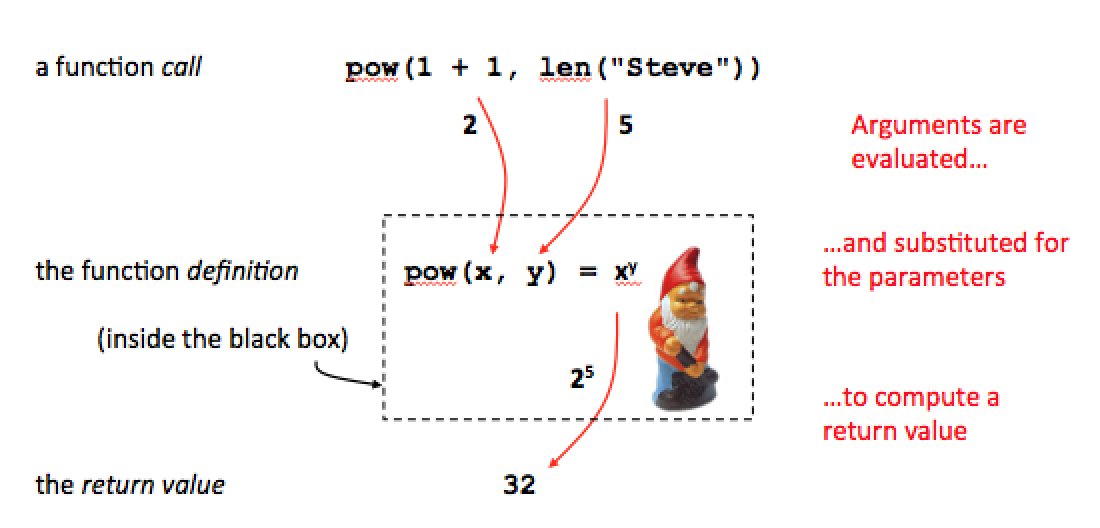So you can see that when we call a function, it really doesn’t matter to us what names were used for the parameters. But inside the function, the function gnome can use those variables in order to produce the result.

## Two kinds of functions¶

We saw in a previous unit that a function in Python can do more than return a value. It can also have side-effects such as printing output. So there are really two kinds of functions:

1.functions that return a value, such as len and pow, and

2.functions that have side-effects (perform some action) but don’t return a value

(Actually, you can also have functions that do both – for example, the function input has side-effects and also return a value. Such functions are not very common in practice, though.)

The first kind – functions that return a value – are the most common and probably the most useful. For example, all the functions built in to your pocket calculator are value-returning functions.

But for our first experiments in writing functions, we will start out writing some simple functions that just print output.

## Be the function gnome!¶

For the first example, let’s write a function that will print one verse to the song, “99 bottles.” Let’s name the function sing_verse. When we call this function, we will provide the current number of bottles, a number between 1 and 99, and the function should print the corresponding verse. Therefore, the function will need one parameter to represent the number of bottles.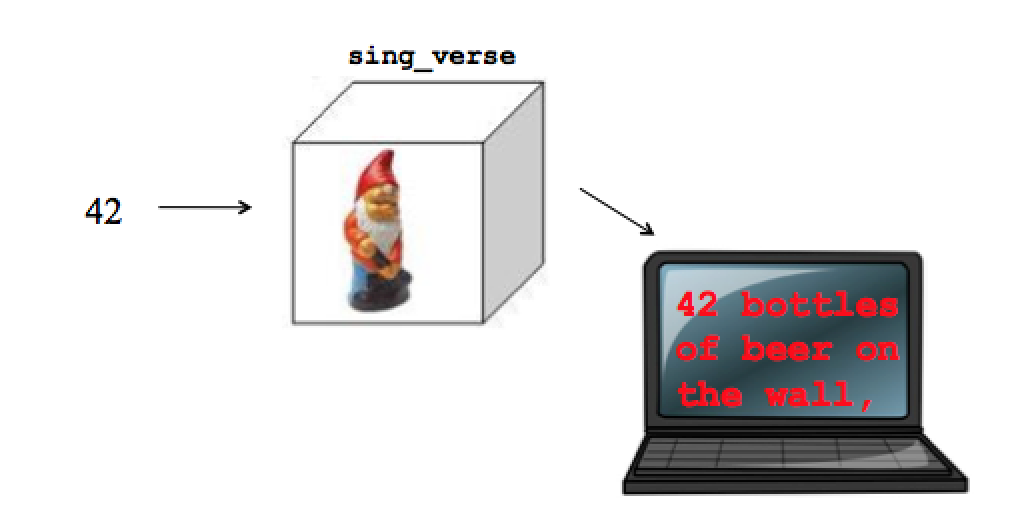A function in Python is defined using the keyword def. The definition of our function will start out like this:

def sing_verse(num):

where we have used a variable called num as the parameter. Next, we have to actually write the statements that will print one verse of the song. These statements form what is called the body of the function. Within the body of the function, we can use the variable num.

Now, if we put this function in a script and run the script, nothing seems to happen. The thing is, we only defined the function. The statements inside the function won’t actually execute until we call the function. For a quick example, let’s just put a couple of function calls in the script right after the function definition:

Each time the function is called, the statements in the function body are executed. The empty print statement just prints a blank line between the two verses.

Let’s go back now and take a careful look at all of the parts of the function definition: We have:

1.the keyword def,

2.the function name,

3.a pair of parentheses containing the parameters,

4.a colon, and

5.a statement block, called the function body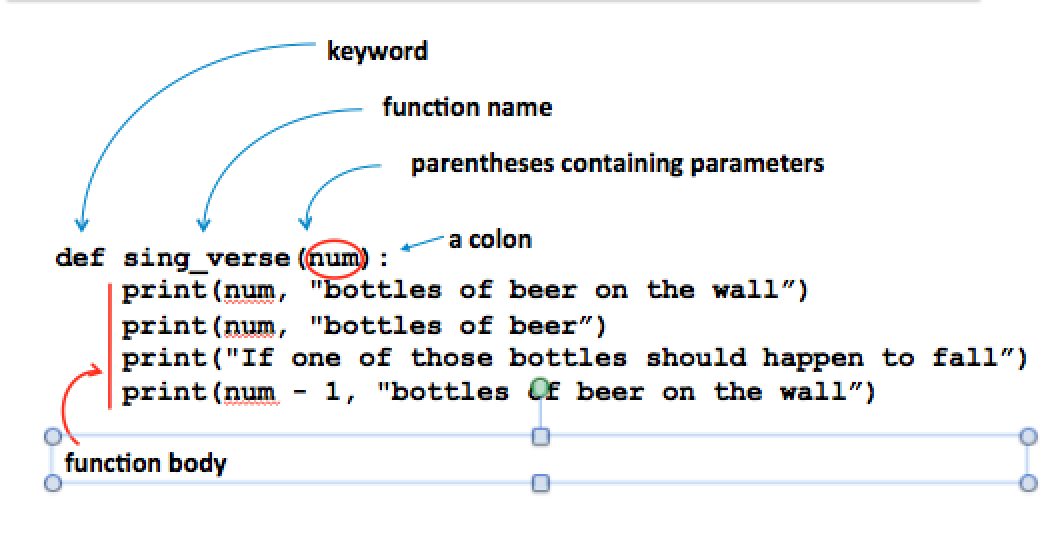Notice that the statements in the body are indented exactly the same amount, just as we saw with the statement blocks in conditional statements. This is important, because it is how the interpreter can distinguish the statements inside the function body from statements that come after the function definition.

Here’s an example with more than one parameter. Let’s write a function that prints the area of a rectangle. In order to compute the answer, we need two values, the length and the width. This tells us we need two parameters for this function. We can use any variable names we want for the parameters, but it might be convenient to call them “length” and “width”. We list them in parentheses after the function name, separated by a comma.

Then when we call the function, we have to supply two arguments, as in this script:

Notice the distinction between

• the parameters to the function, the placeholder variables length and width in the function definition, and
• the arguments to the function, such as the values 5 and 10, that we supply when the function is called

To see their roles, run the code above in codelens. You can see when we execute the statement print_area(5, 10), the two placeholder variables take on the given values within the function body. Try it!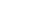It is also possible for a function to have NO parameters. For example, print_cheer is a function that just prints a cheer:Whenever you write a function, the first thing you always have to ask yourself is what the parameters will be. In other words, “what information does this function need in order to do its job?” To print one verse of “99 bottles”, you need to know which verse to sing. To compute the area of a rectangle, you need to know length and the width.

The second thing to ask when you write a function is whether the function should produce output or return a value. So far, all our examples have been functions that produce output. We will write some value-returning functions in the next chapter.

## Modules and importing¶

Why do we write functions? One reason is that after we have gone to the trouble of writing code to perform some task or compute some value, we want to be able to re-use it, just as we were able to re-use the sing_verse function by calling it twice. By putting the code into a function, we can perform the computation again just by calling the function.

But if you look back at the sample scripts we have used so far, you will see a problem. For example, look again at the script in which we defined and used the print_area function: What if we want to use this function in a different script? The problem here is that we are calling the function in the same script in which we’ve defined it. That makes it hard to re-use the function somewhere else. In general, we want to be able to define a function in one script, and make it available to be used in a different script - possibly in many others, if it is a particularly useful function.

Let’s try it. As an experiment, suppose we just take out the call to print_area and put it in a different script. Assume that we have these two files, my_functions.py and test.py, stored in the same directory. Looks all good. But when we run the script test.py, we get a message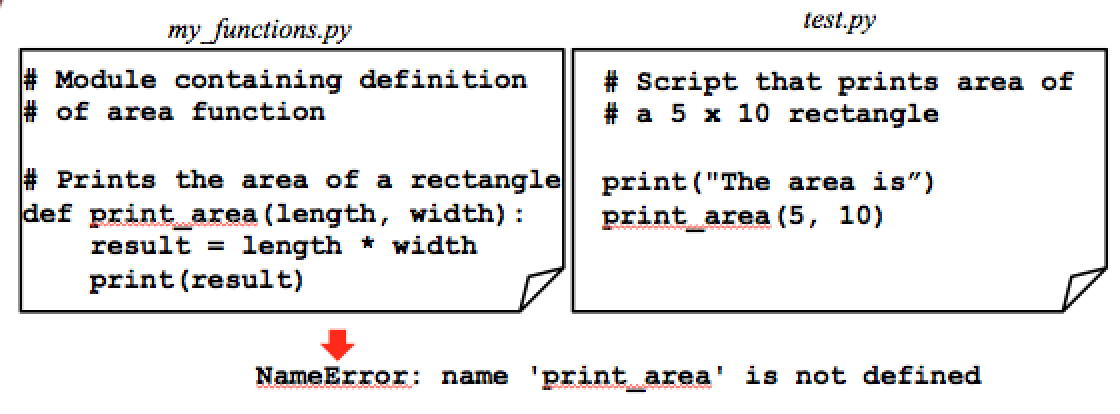What’s up with that?

The thing is, we have to tell the interpreter where to find the print_area function. We do that by putting an import statement at the top of the file test.py: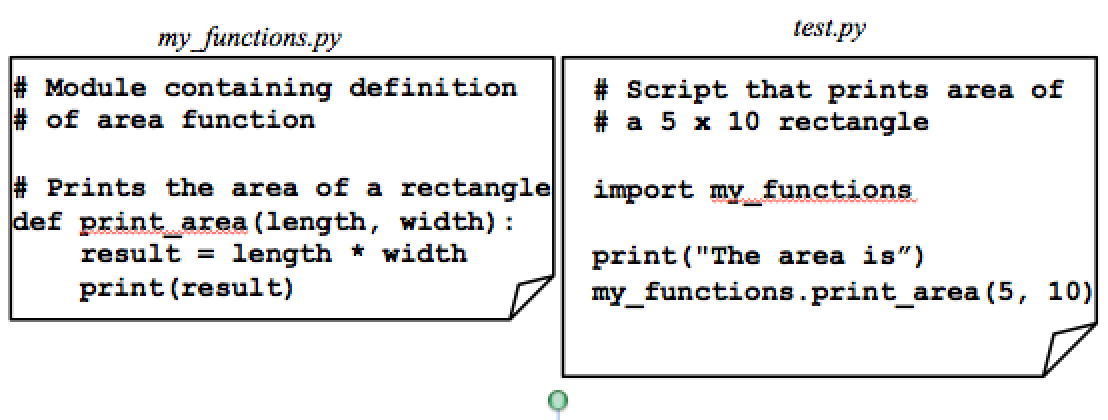The import statement tells the interpreter to look for the function print_area in a module called “my_functions”. What’s a module? We encountered modules before when we used the square root function from the math module. A module is really nothing but a Python script that just contains function definitions. The name of the module is always the file name, without the “.py” extension. For now, we will need to have the module and the script that imports it in the same directory. Later on we will learn some ways to get around this restriction by changing where the interpreter looks for modules.

Next let’s create a user interface for the area function. That is, let’s write a short script that will interact with a user to print the area of any rectangle.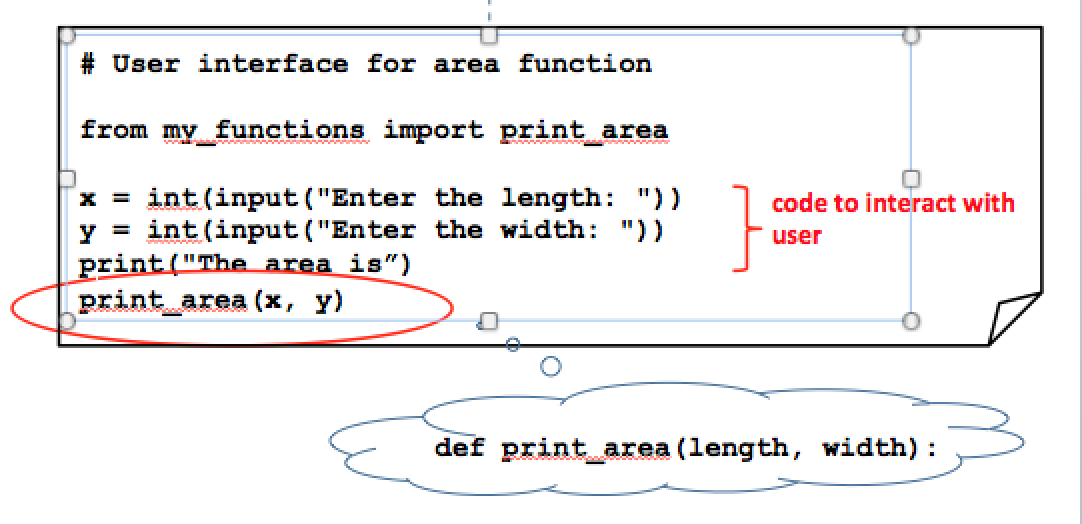## Local variables¶

It is worth noticing that when we write the script above, we can treat the area function as a “black box”. We provide two arguments, namely, the values of the length and width, and the function gnomes work their magic and produce the answer. Notice that we are using variables called “x” and “y” as the arguments to the function. Does that matter? Since we wrote the function ourselves, we may happen to remember that the parameters in the function definition were variables named “length” and “width”, but that makes no difference to us when we call the function. The parameter names only make a difference to us when we’re writing a function.

In fact, NONE of the variables inside a function can ever be seen from outside the function. For example, what happens if we try to directly examine the ‘width’ variable from the function, like this?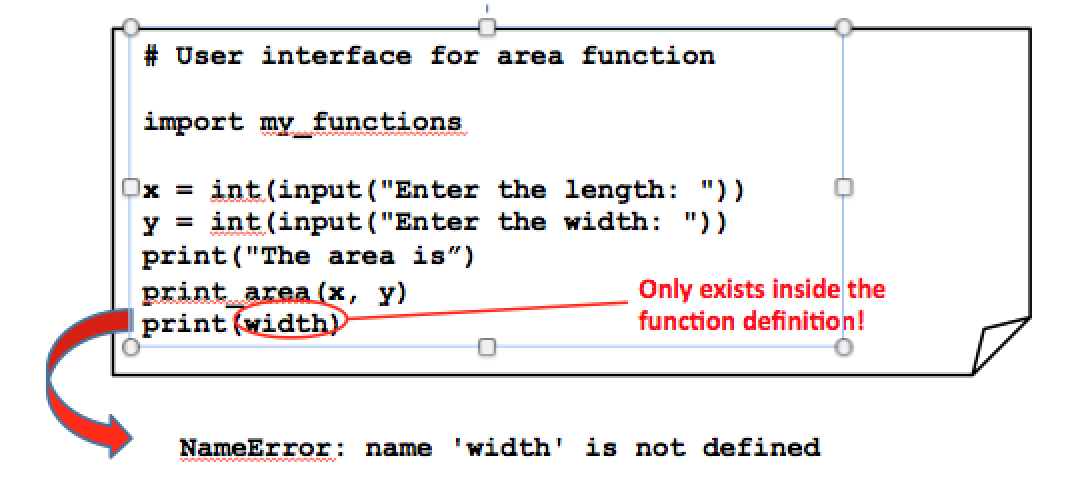The variable ‘width’ is part of the function definition, so we can’t see it from outside the function definition. We say that the variables defined within a function are local variables, since they only exist within the function.

You could say that function definitions are like Vegas:

“What happens in the function definition, stays in the function definition.”

In this unit we have learned to write functions in Python. We will be using functions from now on to keep our work logically organized and to make our code reusable. So far, we have written functions that only have side-effects – that is, functions that produce output. In the next unit we will learn to write value-returning functions too.